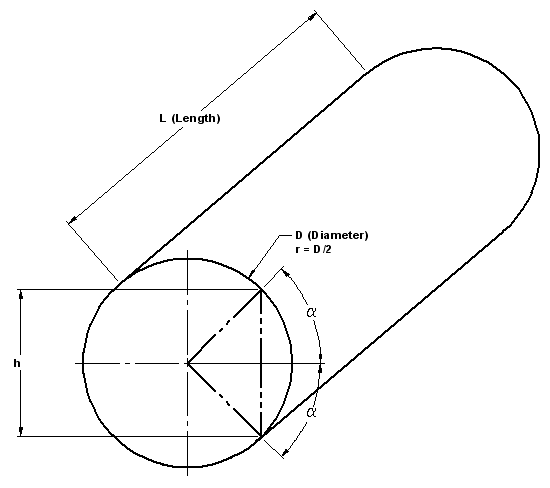Cylinder Stress and Deflection with Applied Torsion

Strength / Mechanics of Materials Menu

The following are the equations and a calculator for determining the cylinder stress and deflection with a known applied torsion or torque. Please note that you must have a java enabled browser. To update the calculated values after changing inputs, you must click "Calculate" located at the bottom of the web page.

Reference: Roark's Formulas for stress and Strain 7th edition Warren C Young - Table 10.1Application Illustration

Shaft Data
r =
mm=
degs
h =Rotational Moment
T =
Nmm
Length under torsion
L =
mm
Young's modulus
E =
N/mm2
Poissons ratio
v =

Modulus of rigidity
G =Torsion constant
C =K =Angular Deflection=Maximum sheer stress (at perimeter)
B ==B =Membership Register | LoginHomeEngineering Book StoreEngineering ForumExcel App. DownloadsOnline Books & ManualsEngineering NewsEngineering VideosEngineering CalculatorsEngineering ToolboxGD&T Training Geometric Dimensioning TolerancingDFM DFA TrainingTraining Online EngineeringAdvertising CenterCopyright Notice